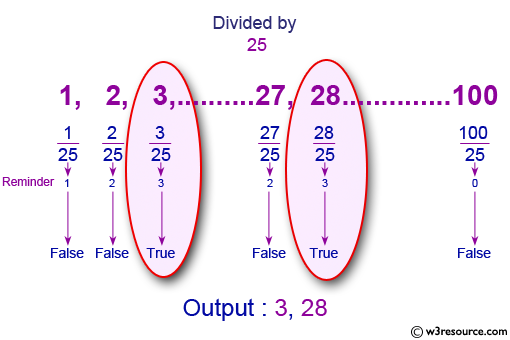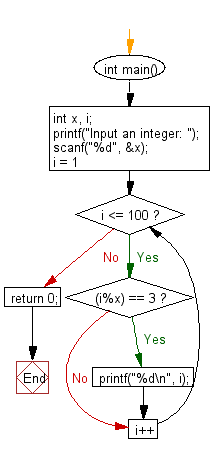﻿ C : Between 1 to 100 divided by 3 will give a remainder of 3

# C Exercises: Print all numbers between 1 to 100 which divided by a specified number and the remainder will be 3

## C Basic Declarations and Expressions: Exercise-32 with Solution

Write a C program to print all numbers between 1 and 100 which are divided by a specified number and the remainder will be 3.

Pictorial Presentation:C Code:

``````#include <stdio.h>
int main() {
int x, i; // Declare variables for user input and loop counter
printf("Input an integer: ");
scanf("%d", &x); // Prompt user for an integer

for(i = 1; i <= 100; i++) // Loop through numbers from 1 to 100
{
if((i%x) == 3) { // Check if the remainder of i divided by x is 3
printf("%d\n", i); // Print i if the condition is met
}
}

return 0;
}
``````

Input data: 25

Sample Output:

```Input an integer: 3
28
53
78
```

Flowchart:C Programming Code Editor:

What is the difficulty level of this exercise?

Test your Programming skills with w3resource's quiz.

﻿